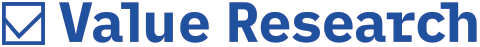Calculating NAV

The net asset value of a mutual fund is calculated on the basis of its daily price change

How is the net asset value of a mutual fund calculated?
-AK Sidhantu

Think of the net asset value of a mutual fund as a price. Every day, when you give your money to a mutual fund, the fund invests that money into a range of securities. Those securities trade in the market and close at a certain value at the end of the day. The propitiate ownership of that fund is calculated and divided by the total number of units. So, the net asset value is calculated on the basis of the change in price. Assuming that you are buying a Sensex index fund and the Sensex goes up by 1 per cent, then the fund’s net asset value will go up by 1 per cent, less the expenses incurred by the fund on that day.